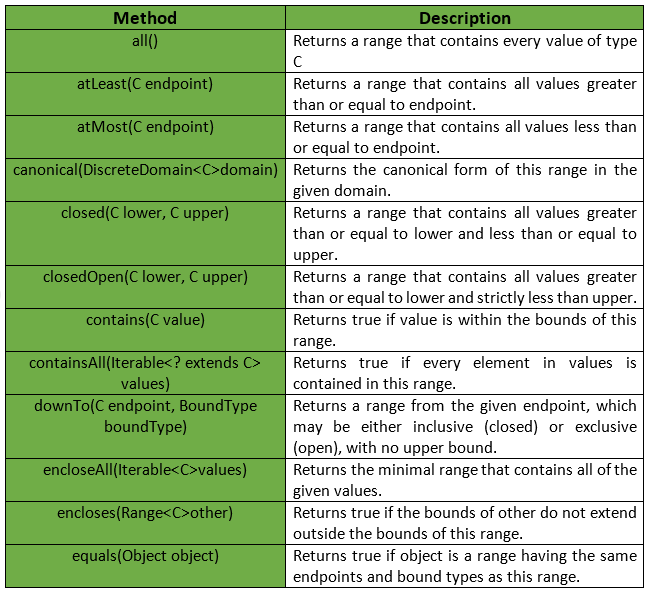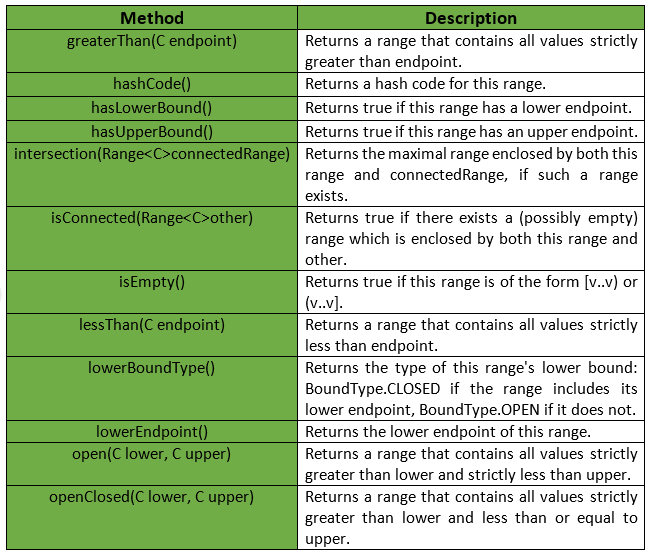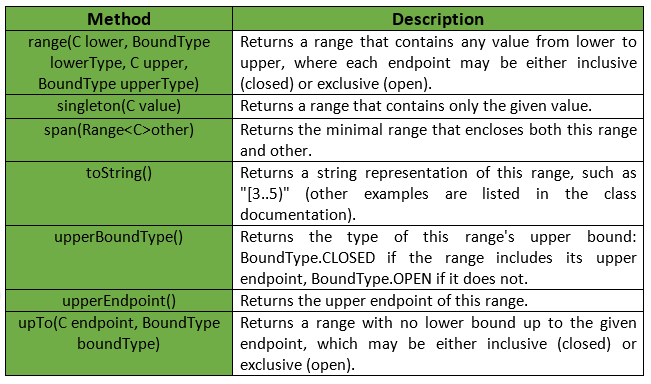# Range Class | Guava | Java

Guava’s Range represents an interval, for example, a < range < b. Here range includes any value between a and b, called endpoints which form the boundary. Any value between the boundary is a contiguous span of values of type Comparable.

Declaration : The declaration for com.google.common.collect.Range<C> class is :

```@GwtCompatible
public final class Range<C extends Comparable>
extends Object
implements Predicate<C>, Serializable
```

Each end of the range may be bounded or unbounded. If bounded, there is an associated endpoint value. If not it will be treated as infinity. A range can be further defined as either open or closed based whether the range is exclusive or inclusive of the endpoints.

• open(a, b) : It represents a < range < b, and in notation form, (a, b).
• closed(a, b) : It represents a <= range <= b, and in notation form, [a, b].
• openClosed(a, b) : It represents a < range <= b, and in notation form, (a, b].
• closedOpen(a, b) : It represents a <= range < b, and in notation form, [a, b).
• greaterThan(a) : It represents range > a, and in notation form, (a..+inf).
• atLeast(a, b) : It represents range >= a, and in notation form, [a..+inf).
• lessThan(a, b) : It represents range < b, and in notation form, (-inf..b).
• atMost(a, b) : It represents range <= b, and in notation form, (-inf..b].
• all() : It represents -inf < range < +inf, and in notation form, (-inf..+inf).

Below given are some methods provided by Range Class of Guava :Note : When both endpoints exist, the upper endpoint may not be less than the lower. The endpoints may be equal only if at least one of the bounds is closed :

• [a..a] : a singleton range.
• [a..a) or (a..a] : empty ranges, also valid.
• (a..a) : invalid, an exception will be thrown.

Some other methods provided by Range Class of Guava are :Exceptions :

• open : IllegalArgumentException if lower is greater than or equal to upper.
• closed : IllegalArgumentException if lower is greater than upper.
• closedOpen: IllegalArgumentException if lower is greater than upper.
• openClosed : IllegalArgumentException if lower is greater than upper.
• range : IllegalArgumentException if lower is greater than upper.
• encloseAll : ClassCastException if the parameters are not mutually comparable, NoSuchElementException if values is empty, NullPointerException if any of values is null.
• lowerEndpoint : IllegalStateException if this range is unbounded below (that is, hasLowerBound() returns false).
• lowerBoundType : IllegalStateException if this range is unbounded below (that is, hasLowerBound() returns false).
• upperEndpoint : IllegalStateException if this range is unbounded above (that is, hasUpperBound() returns false).
• upperBoundType : IllegalStateException if this range is unbounded above (that is, hasUpperBound() returns false).
• intersection : IllegalArgumentException if isConnected(connectedRange) is false.Below given are some examples to understand the implementation in a better way :
Example 1 :

 `// Java code to show implementation ` `// of Range class of Guava ` `import` `com.google.common.collect.Range; ` ` `  `class` `GFG { ` ` `  `    ``// Driver code ` `    ``public` `static` `void` `main(String[] args) ` `    ``{ ` ` `  `        ``// Taking range (1, 5) ` `        ``// Note that here 1 and 5 are not included ` `        ``Range range = Range.open(``1``, ``5``); ` ` `  `        ``// Checking if range contains 1 or not ` `        ``System.out.println(range.contains(``1``)); ` ` `  `        ``// Checking if range contains 2 or not ` `        ``System.out.println(range.contains(``2``)); ` ` `  `        ``// Checking if range contains 3 or not ` `        ``System.out.println(range.contains(``3``)); ` ` `  `        ``// Checking if range contains 4 or not ` `        ``System.out.println(range.contains(``4``)); ` `    ``} ` `} `

Output :

```false
true
true
true
```

Example 2 :

 `// Java code to show implementation ` `// of Range class of Guava ` `import` `com.google.common.collect.Range; ` ` `  `class` `GFG { ` ` `  `    ``// Driver code ` `    ``public` `static` `void` `main(String[] args) ` `    ``{ ` ` `  `        ``// Taking range [1, 5] ` `        ``// Note that here 1 and 5 are included ` `        ``Range range = Range.closed(``1``, ``5``); ` ` `  `        ``// Checking if range contains 1 or not ` `        ``System.out.println(range.contains(``1``)); ` ` `  `        ``// Checking if range contains 2 or not ` `        ``System.out.println(range.contains(``2``)); ` ` `  `        ``// Checking if range contains 3 or not ` `        ``System.out.println(range.contains(``3``)); ` ` `  `        ``// Checking if range contains 5 or not ` `        ``System.out.println(range.contains(``5``)); ` `    ``} ` `} `

Output :

```true
true
true
true
```

Example 3 :

 `// Java code to show implementation ` `// of Range class of Guava ` `import` `com.google.common.collect.Range; ` ` `  `class` `GFG { ` ` `  `    ``// Driver code ` `    ``public` `static` `void` `main(String[] args) ` `    ``{ ` ` `  `        ``// Taking range (2, +inf) ` `        ``// Note that numbers less than equal to 2 ` `        ``// are not included ` `        ``Range range = Range.greaterThan(``2``); ` ` `  `        ``// Checking if range contains 1 or not ` `        ``System.out.println(range.contains(``1``)); ` ` `  `        ``// Checking if range contains 2 or not ` `        ``System.out.println(range.contains(``2``)); ` ` `  `        ``// Checking if range contains 130 or not ` `        ``System.out.println(range.contains(``130``)); ` ` `  `        ``// Checking if range contains 500 or not ` `        ``System.out.println(range.contains(``500``)); ` `    ``} ` `} `

Output :

```false
false
true
true
```

Example 4 :

 `// Java code to show implementation ` `// of Range class of Guava ` `import` `com.google.common.collect.Range; ` ` `  `class` `GFG { ` ` `  `    ``// Driver code ` `    ``public` `static` `void` `main(String[] args) ` `    ``{ ` ` `  `        ``// Taking range (-inf, 2] ` `        ``// Note that only numbers less than equal to 2 ` `        ``// are included ` `        ``Range range = Range.atMost(``2``); ` ` `  `        ``// Checking if range contains 1 or not ` `        ``System.out.println(range.contains(``1``)); ` ` `  `        ``// Checking if range contains 2 or not ` `        ``System.out.println(range.contains(``2``)); ` ` `  `        ``// Checking if range contains -1 or not ` `        ``System.out.println(range.contains(-``1``)); ` ` `  `        ``// Checking if range contains 5 or not ` `        ``System.out.println(range.contains(``5``)); ` `    ``} ` `} `

Output :

```true
true
true
false
```

Attention reader! Don’t stop learning now. Get hold of all the important Java and Collections concepts with the Fundamentals of Java and Java Collections Course at a student-friendly price and become industry ready.

My Personal Notes arrow_drop_upIn love with a semicolon because sometimes i miss it so badly)

If you like GeeksforGeeks and would like to contribute, you can also write an article using contribute.geeksforgeeks.org or mail your article to contribute@geeksforgeeks.org. See your article appearing on the GeeksforGeeks main page and help other Geeks.

Please Improve this article if you find anything incorrect by clicking on the "Improve Article" button below.

Article Tags :
Practice Tags :

Be the First to upvote.

Please write to us at contribute@geeksforgeeks.org to report any issue with the above content.